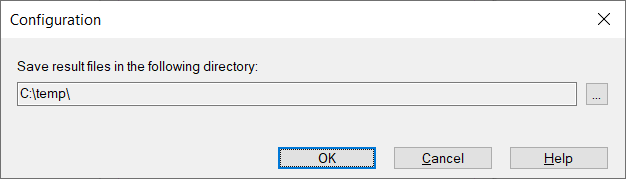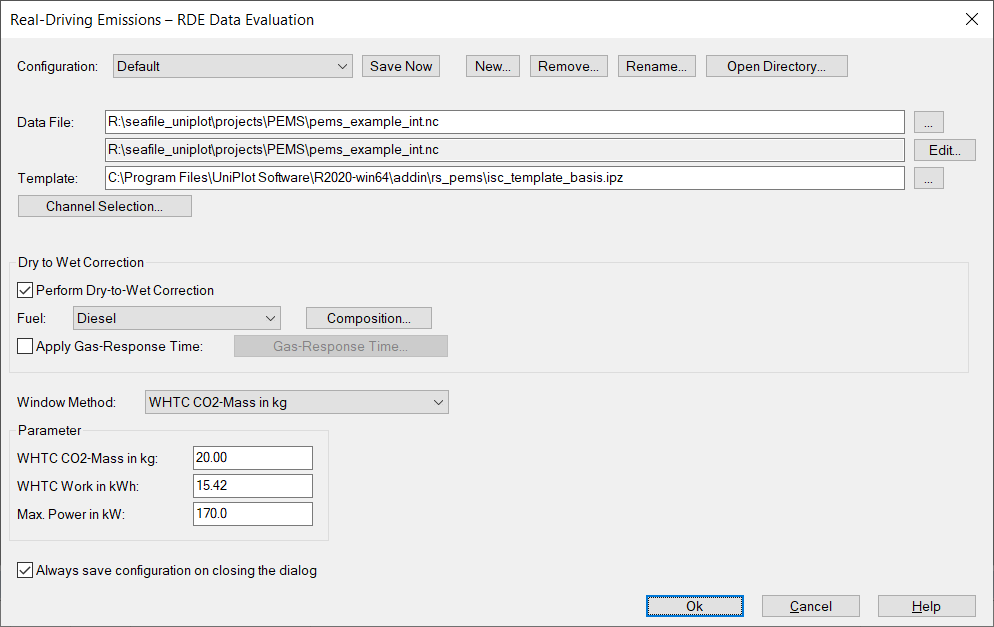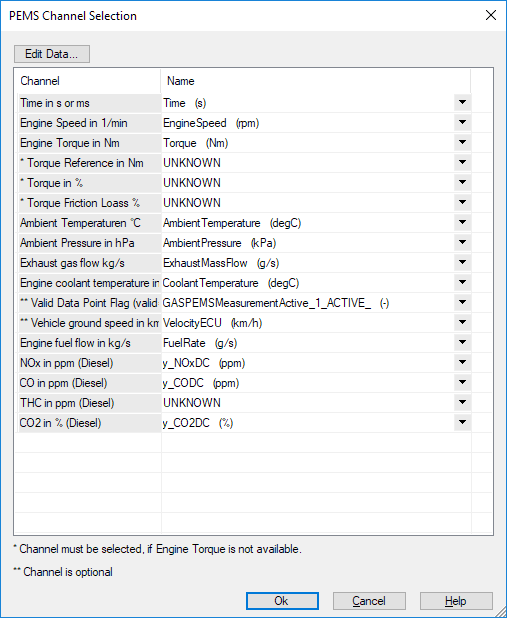# Real Driving Emission Data Evaluation¶

Note

The addin is a beta version. The release version is planned for 2021.

The Addin calculates the emission conformity factor from data measured by a PEMS systen during an on-the-road test. A dry/wet correction after ISO 16183:2002(E) and a gas delay correction can be applied to the data. The data from the pems device and the ECU must be time aligned and merged into one file.

The following channels are mandatory:

Name Alias Unit
Time time_s s
Engine Speed speed_rpm 1/min
Engine Torque torque_nm Nm
Torque Reference Torque_Reference Nm
Torque Percent Torque_Percent %
Torque Friction Loss Torque_Friction %
Ambient Temperature ambient_temperature_grdC °C
Ambient Pressure ambient_pressure_hpa hPa
Exhaust gas flow ExhaustMassFlow__kgs kg/s
Engine coolant temperature CoolantTemperature °C
Fuel Mass Flow fuel_mass_flow_kg_s kg/s

The following channels are optional:

Name Alias Unit
Valid Data Point Flag (valid=1) ValidPoint 0/1-Signal
Vehicle Speed VehicleSpeed km/h

The Addin uses the method “Moving Averaging Window Principle” (as used by EMROAD) to determine the conformity factors. The emission data is integrated using a moving average window, based on the reference engine work or CO2 mass.

The data must be measured with a frequency of at least 1 Hz. If the data was measured with a higher frequency, it will be resampled to 1 Hz. Data in time gaps will be set to zero and ignored by the MAW calculation.

## Dry/Wet correction ISO 16183:2002(E)¶

The `Dry/wet correction` in the main dialog box is executed during the data import. Da dialog box will be displayed to select the mandatory channels.

## Test Data Verification¶

The environment temperature is checked against the lower limit of -7 °C and the upper limit.

The data evaluation will start after the coolant temperature has reached 30 °C for the first time or after the coolant temperature is stabilised within +/– 2°C over a period of 5 minutes whichever comes first but no later than 10 minutes after engine start.

The valid windows are the windows whose average power exceeds the power threshold of 20 % of the maximum engine power. It is checked, if the percentage of valid windows is equal or greater than 50 %.

## Usage¶

• Start UniPlot.
• Choose RDE Evaluation=>Configuration to select emission channels:• Choose ISC Evaluation=>Evalution to start a new evalutation:## Data Selection¶

The data can be imported from all supported data formats. The unit converter is used to convert the units used for the RDE calculation.

## Channel Selection¶

The channels mandatory for the calculation can be selected using the following dialog box:## Calculation¶

All calculated results will be written into the result data file. Its name ist displayed in the Event Window. A double click loads the file into the data browser.

The following channels will be added to the data file:

Name Unit Description
e.Trip_Work_int kWh Total trip work.
e.Trip_Work_int_a kWh Total trip work of valid data points.
e.AmbientTemp_max_limit °C Calculated maximum valid ambient temperature.
e.MAW_Time s Lenght of time of each moving average window.
e.MAW_Power_percent % Percent of each moving average window of the specified max. power.
e.MAW_start_time s Start time of each moving average window.
e.MAW_end_time s End time of each moving average window.
e.MAW_duration_time s Length of each moving average window
e.MAW_invalid_points   Number of invalid points in each moving average window.
e.MAW_XXX g/kWh or #/kWh Emission values for each moving average window.
e.CF_all_XXX   Conformity factor for each moving average window for all emissions.
e.CF_XXX_Distribution_y   Sorted conformity factor for each moving average window for all emissions.
e.CF_XXX_Distribution_y_unsorted   Unsorted conformity factor for each moving average window for all emissions.
e.CF_Distribution_x % X-Coordinates for `e.CF_XXX_Distribution_y` and `e.CF_XXX_Distribution_y_unsorted`.
e.vehicle_speed km/h Vehicle velocity
e.Trip_Cummulativ km trip distance cummulativ
e.Trip_Fuel_Consumption g Fuel consumption for the trip
e.XXX ppm or # Copy of specified emission channel.
e.Trip_flow_XXX #/s Particle number
e.Trip_int_XXX # Particle number integral.
e.Trip_mass_flow_XXX g/s Emission mass.
e.Trip_mass_int_XXX g Emission mass integral.
e.torque Nm Torque channel (copy of the specified channel).
e.Time s time channel (copy of the specified channel).
e.speed 1/min engine speed (copy of the specified channel).
e.exhaust_mass_flow kg/s exhaust mass flow (copy of the specified channel).
e.coolant_t °C Coolant temperatur (copy of the specified channel).
e.ambient_t °C Ambient temperatur (copy of the specified channel).
e.ambient_p kPa Ambient Pressure (copy of the specified channel).
e.valid   Valid points (0 or 1) (copy of the specified channel).
e.Power kW Calculated power
e.Power_percent % Power in percent (power / power_max * 100).

The following attribute values will be added to the data file:

(XXX stands for emission channel)

Name Unit Description
Name Einheit Beschreibung
e.work_window_limit kWh Reference Work, specified in the main dialog box.
e.coolant_min_limit °C Minimum coolant temperature (Default: -7 °C).
e.power_max kW Maximum Power, specified in the main dialog box.
e.percentile % Percentile value.
e.power_limit_percent % Moving Average Percentil for which the emission values are calculated.
e.ugas_XXX   UGas values for the different emissions (XXX = NOx, CO2, etc.)
e.limit_XXX Unit of XXX Limits for the given emissions Test cycle (WHTC). (XXX = NOx, CO2, etc.)
e.Trip_Duration s Tripe Time for the complete trip from engine start to stop (engine speed > 100 rpm at the beginning and end of test).
e.Trip_AV_Speed 1/min Average engine speed for the complete trip.
e.Trip_AV_Torque Nm Average torque for the complete trip.
e.Trip_AV_Power kW Average power for the complete trip.
e.Trip_Work kWh Work for the complete trip.
e.Trip_Work_a kWh Work for the complete trip for all valid points (_a: calibration during test is ignored).
e.AmbientTemp_invalid_points   Number of invalid points, (T_ambient < Tmin OR T_ambient > Tmin).
e.AmbientTemp_min_limit °C Ambient minimum temperature (T_min), Tmax is calculated: -0.4514 .* (101.3 - rvAP) + (311-273.15)), rvAP is the ambient pressure in kPa.
e.MAW_Work kWh Work of all moving average windows
e.MAW_Duration s Time of all moving average windows.
e.Trip_MAW_AV_speed 1/min Average engine speed for all moving average windows.
e.Trip_MAW_AV_torque Nm Average engine speed for all moving average windows.
e.Trip_MAW_AV_power kW Average power for all moving average windows.
e.Trip_MAW_AV_coolant_t °C Average coolant temperature for all moving average windows.
e.Trip_MAW_AV_ambient_t °C Average ambient temperature for all moving average windows.
e.Trip_MAW_AV_ambient_p kPa Average ambient pressure for all moving average windows.
e.Trip_MAW_AV_XXX Unit of XXX Average emission for all moving average windows. (XXX = NOx, CO2, etc.)
e.Q90_XXX g or #/kWh for particle in window Quantile value for emission XXX at the specified percentil value, default value 90%. (e.percentile)
e.CF_Q90_XXX   Conformity factor for emission XXX at the specified percentil value.
e.MAW_valid_windows   Number of valid windows.
e.MAW_all_windows   Number of windows.
e.MAW_T70_Windows   Number of windows where coolant temperatur is above 70 °C.
e.MAW_valid_windows_percent % Number of valid windows in percent (nValidWindows/nWindows * 100).
e.MAW_valid_Power_Min_percent % minimum power of all valid windows.
e.MAW_valid_Power_Max_percent % maximum power of all valid windows.
e.speed_limit_zero km/h vehicle velocity limit for zero velocity (Default: 2 km/h)
e.speed_limit_urban km/h vehicle speed limit for urban driving (Default: 50 km/h)
e.speed_limit_interurban km/h vehicle speed limit for inter urban driving (Default: 75 km/h)
e.Trip_Zero % Percent of trip time with zero vehicle velocity.
e.Trip_Urban % Percent of trip time with urban vehicle velocity.
e.Trip_InterUrban % Percent of trip time with inter urban vehicle velocity.
e.Trip_Highway % Percent of trip time with highway vehicle velocity (v > v_inter_urban).
e.Trip_Velocity_Mean km/h Average vehicle velocity.
e.Trip_Vehicley_Max km/h Maximum vehicle velocity for the complete trip.
e.Trip_Distance km Trip distance.
e.Trip_Fuel_Mass g complete fuel consumption for the trip.
e.Trip_bsfc g/kWh Specific fuel consumption for the trip.
e.ColdStartDuration s Time at the test start until the coolant temperatur is valid.
e.Trip_AV_XXX Unit of XXX Average of all valid data points.
e.Trip_total_XXX ppm of # Total emissions for the trip.
e.Trip_spez_XXX” g/kWh or #/kWh Specific emission for alle valid values (a).
e.Trip_spez_distance_XXX g/km or #/km Distance specific emission for all valid values (a).
e.Trip_spez_fuel_XXX g/kg or #/kg Fuel consumption specific emission for all valid values (a).
e.Trip_mass_XXX g Complete emission mass. (XXX = NOx, CO2, etc.)
e.channel.XXX   Emission channel name.
e.Trip_Duration s Tripe Time for the complete trip from engine start to engine stop (engine speed > 100 rpm).

## Template¶

The template will be filled with the data from the selected datafile.

The result file contains all data of the selected file and the result data. The result data and attributes starts with the prefix `e.`. If global attributes from the NC file are used to be displayed in the IPZ document via placeholder, the period (.) must be replaced by an underscore.

```Attribute: e.CF_Final_NOx --> Placeholder: \$e_CF_Final_NOx\$
```

An example file can be found in the addin rs_pems folder.

## Programming¶

The source code can be found in `<uniplot>\\addin\rs_pems`:

```def _pems_calculate_test_example1()
{
obj = _pems_create();

obj.ssNCFile = "s:/projects/pems/pems_example.nc2"
if (obj.ssNCFile == "") {
return FALSE;
}
obj.rsWork_Ref_kWh = 30;
obj.rsCoolant_Limit_grdC = 70;
obj.rsPower_Max_kW = 250;
obj.rsPower_Ref_percent = 20;
obj.rsPercentile = 90;
obj.uGasValues.NOx = 0.001587;
obj.limit.Nox = 0.46;

obj.oVar["time_s"] = "Time";
obj.oVar["speed_rpm"] = "iENG_SPEED";
obj.oVar["torque_nm"] = "";
obj.oVar["Torque_Reference"] = "sREF_ENG_TORQ";
obj.oVar["Torque_Percent"] = "iPCNT_TORQUE";
obj.oVar["Torque_Friction"] = "iFRICT_TORQUE";
obj.oVar["CoolantTemperature"] = "iCOOL_TEMP";
obj.oVar["NOx"] = "ikNOxzw";
obj.oVar["ExhaustMassFlow__kgs"] = "icMASS_FLOW";
obj.oVar["ambient_temperature_grdC"] = "iSCB_LAT";
obj.oVar["ambient_pressure_hpa"] = "iSCB_LAP";

return _pems_calculate_main(obj);
}
```

History

Version Description
R2017.0 New.
R2020.6 (0.2.5) The evaluation has been adapted to “COMMISSION REGULATION (EU) 2019/1939 of 7 November 2019” The avarage value windows are now determined in forward direction. The start of the evaluation is adjusted according to the regulation 2019/1939. The CF factor is calculated from the cold phase and hot phase in the ratio 14 to 86. The dialog box has been modified: The windows can now be calculated using the work or the cumulated CO2 mass emission.

id-1162246